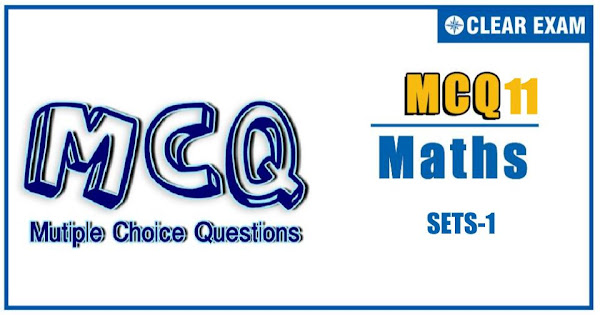## SETS-1 Quiz-11

In mathematics a set is a collection of distinct elements. The elements that make up a set can be any kind of things: people, letters of the alphabet, numbers, points in space, lines, other geometrical shapes, variables, or even other sets. Two sets are equal if and only if they have precisely the same elements. Sets are ubiquitous in modern mathematics. Indeed, set theory, more specifically Zermelo–Fraenkel set theory, has been the standard way to provide rigorous foundations for all branches of mathematics since the first half of the 20th century..

Q1. The value of (A∪B∪C) 〖∩(A∩B^C∩C^C )〗^C 〖∩C〗^Cis
•  B∩C^C
•  B^C∩C^C
•  B∩C
•  A∩B∩C
Q2.Let n be a fixed positive integer. Define a relation R on the set Z of integers by, a R b⇔n | a-b. Then, R is not
•  Reflexive
•  Symmetric >
•   Transitive
•  None of these
Solution
D

Q3.  Let R and S be two equivalence relations on a set A. Then,
•   R∪S is an equivalence relation on A
•  R∩S is an equivalence relation on A
•  R-S is an equivalence relation on A
•  None of these
Solution
B

Q4. Let A be the set of all students in a school. A relation R is defined on A as follows: "aRb iff a and b have the same teacher”
•  Reflexive
•  Symmetric
•  Transitive
•  Equivalence
Solution
(d) We have, n(A∩B ̅ )=9,n(A ̅∩B)=10 and n(A∪B)=24 ⇒n(A)-n(A∩B)=9,n(B)-n(A∩B)=10 and, n(A)+n(B)-n(A∩B)=24 ⇒n(A)+n(B)-2n(A∩B)=19 and n(A)+n(B)-n(A∩B)=24 ⇒n(A∩B)=5 ∴n(A)=14 and n(B)=15 Hence, n(A×B)=14×15=210

Q5.Let R be an equivalence relation on a finite set A having n elements. Then, the number of ordered pairs in R is
•  Less than n
•  Greater than or equal to n
•  Less than or equal to n
•  None of these
Solution
(b) Since R is an equivalence relation on set A. Therefore (a,a)∈R for all a∈A. Hence, R has at least n ordered pairs

Q6. Consider the following statements: p∶ Every reflexive relation is symmetric relation q∶ Every anti-symmetric relation is reflexive Which of the following is/are true?
•  p alone
•  q alone
• Both p and q
•  Neither p nor q
Solution
(d) Clearly, none of the statements is true

Q7. If P is the set of all parallelograms, and T is the set of all trapeziums, then P∩T is
•  P
•  T
•  ϕ
•  None of these
Solution
(a) Clearly, P⊂T ∴P∩T=P

Q8. The relation R={(1,1),(2,2),(3,3),(1,2),(2,3),(1,3)} on set A={1,2,3} is
•  Reflexive but not symmetric
•  TReflexive but not transitive
•  Symmetric and transitive
•  Neither symmetric nor transitive
Solution
(a) Since (1,1),(2,2),(3,3)∈R. Therefore, R is reflexive. We observe that (1,2)∈R but (2,1)∉R, therefore R is not symmetric. It can be easily seen that R is transitive

Q9. If two sets A and B are having 99 elements in common, then the number of elements common to each of the sets A×B and B×A are
•  2^99
•  99^2
•  100
•  18
Solution
(b) Number of elements common to each set is 99×99=99^2.

Q10. A survey shows that 63% of the Americans like cheese whereas 76% like apples. If x% of the Americans like both cheese and apples, then
•  x=39
•  x=63
•  39≤x≤63
• None of these
Solution
(c) Given, n(C)=63,n(A)=76 and n(C∩A)=x We know that, n(C∪A)=n(C)+n(A)-n(C∩A) ⇒ 100=63+76-x⇒x=139-100=39 And n(C∩A)≤n(C) ⇒ x≤63 ∴39≤x≤63#### Written by: AUTHORNAME

AUTHORDESCRIPTION## Want to know more

Please fill in the details below:

## Latest NEET Articles\$type=three\$c=3\$author=hide\$comment=hide\$rm=hide\$date=hide\$snippet=hide

Name

ltr
item
BEST NEET COACHING CENTER | BEST IIT JEE COACHING INSTITUTE | BEST NEET & IIT JEE COACHING: Sets-1-Quiz-11
Sets-1-Quiz-11
https://1.bp.blogspot.com/--z896mlkDRg/YOP_unVRDdI/AAAAAAAAJAA/1gEbTlXuscMYn_kk9vGa08E4NZy1jfZawCLcBGAsYHQ/s600/Quiz%2BImage%2B20.jpg
https://1.bp.blogspot.com/--z896mlkDRg/YOP_unVRDdI/AAAAAAAAJAA/1gEbTlXuscMYn_kk9vGa08E4NZy1jfZawCLcBGAsYHQ/s72-c/Quiz%2BImage%2B20.jpg
BEST NEET COACHING CENTER | BEST IIT JEE COACHING INSTITUTE | BEST NEET & IIT JEE COACHING
https://www.cleariitmedical.com/2021/07/sets-1-quiz-11.html
https://www.cleariitmedical.com/
https://www.cleariitmedical.com/
https://www.cleariitmedical.com/2021/07/sets-1-quiz-11.html
true
7783647550433378923
UTF-8

STAY CONNECTED